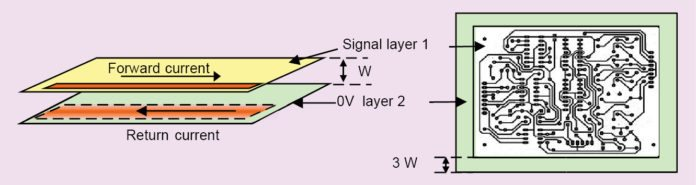# Electromagnetic Compatibility: Multi-Layer PCB Designing (Part 2 of 5)In the first part we touched base on multi-layer PCB design. In this part we discuss more about the same.

## Multi-layer board and high-speed PCB design

A multi-layer board is a PCB having separate layers for power, ground and circuit tracks. It facilitates the use of entire copper layers for power and ground connections called power planes and ground planes, respectively. This decreases their inductance and impedance, allowing the use of cb high clock and signal frequencies and reducing ground noise. A rule of thumb is that, all other things being equal, a four-layer board will produce 15dB less radiation than a two-layer board.

New techniques employed in PCB design and manufacturing, on one hand, made it possible to drastically reduce the size of the PCB. On the other hand, however, clock frequencies have also increased dramatically, resulting in very fast rise times (or short edge rates) of clocks. Such short edge rates mean that decreased PCB dimensions have started to have significant effects on signal voltages and currents.

High-speed circuits are those circuits that use clocks of fast (or small) rise times. But how does one define high speed? Well, the term high speed is relative, where one is essentially comparing two speeds. Take clocks, for example. One of these two speeds is the rise time of the clocks, while the other is the speed of propagation of the electrons of the current that constitutes the clocks.

Now, the speed of movement of electrons in a PCB trace is less that the speed through air (which is the speed of light) since the field surrounding the electron has to travel through the PCB material that creates a drag. The equation given below gives the relation between speed of wave Vw along a PCB trace surrounded by PCB material to its speed C through a trace surrounded by air. er is relative permittivity of the PCB material.

### Vw = C/√er

For example, speed of an electromagnetic field in a trace surrounded by air is about 30.5cm (12-inch) per nanosecond (hs). But when the trace is laid on an epoxy PCB (with er of 4), speed decreases to 15.2cm (6-inch) per nanosecond.

Now, consider a clock whose rise time is 1hs, and if dimensions of a PCB are greater than half the length traversed by this clock in 1hs (that is, greater than 7.6cm or 3-inch), track lengths will start to have significant effects on voltages. Let us see how.

Fig. 6 shows a typical clock with rise time of 1hs. In this time, current associated with this clock will travel 15.2cm (6-inch) along the track. Now, if the PCB dimension is less than 7.6cm (3-inch), reflected wave (wave will be invariably reflected if track changes direction or there is increase or decrease in track width) will arrive in less than 1hs (point X in Fig. 6), that is, at a time when the driver is still ramping the pulse up into the trace.

Because of driver inertia, reflected pulse will not have any effect on incident pulse. But if PCB dimensions (or track discontinuities) are greater than 7.6cm (3-inch), reflected pulse will arrive at point Y. Since driven clock is now stable, reflected pulse will have significant effect on incident clock.

Hence, for a PCB with dimensions greater than 7.6cm (3-inch), clock with rise times of 1hs will be considered high-speed and, for such cases, a radical design approach termed as high-speed PCB design using multi-layer boards will have to be followed.

## Multi-layer concepts in Multi-Layer PCB Design

Let us take a look at some basic terms related to multi-player boards that we will be referring to time and again.

Board or layer stack-up. Stack-up is the name given to the order of various etched copper and dielectric layers that make up the board.

Power and ground planes. Multi-layer boards invariably use two layers of un-etched copper that are in the form of a continuous sheet, one for power supply (Vcc) and the other for ground (return), which are normally referred to as power and ground planes. Use of individual planes for power and ground lowers power distribution impedance and also reduces the signal-loop area. Hence, compared to a two-layer PCB, a simple four-layer PCB using such signal distribution can provide approximately 30dB improvement in EMC performance.

## Reducing fringing fields: The 20H rule

Radio frequency (RF) currents between power and ground planes will cause emissions at the edges of planes. Such emissions are sometimes referred to as edge-fired emissions or fringing fields. These can be reduced by reducing the size of the power plane with respect to ground plane.

Ground plane dimensions [on layer 3 in Fig. 7(a)] should exceed power plane dimensions by 20H on all four sides, where H is the distance between power and ground planes. This will increase intrinsic self-resonant frequency of the PCB. Decreasing spacing between 0V and power planes also reduces fringing fields. Further decrease can be achieved by using a decap wall around the periphery of the power plane and inside the 0V plane periphery by 20H, where H is the plane pair spacing.1.anonymous